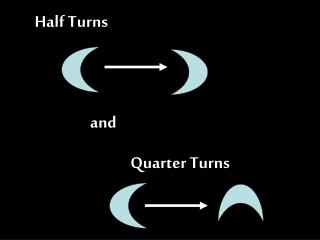DownloadDownload PresentationHalf Turns

# Half Turns

Télécharger la présentation## Half Turns

- - - - - - - - - - - - - - - - - - - - - - - - - - - E N D - - - - - - - - - - - - - - - - - - - - - - - - - - -
##### Presentation Transcript

1. Half Turns and Quarter Turns

2. This house is the right way up.

3. This house has been turned a half turn

4. This house is the right way up.

5. This house has been turned a quarter turn to the right

6. This house is the right way up.

7. This house has been turned a quarter turn to the left.

8. This heart is the right way up.

9. How has this heart been turned? A quarter turn to the right.

10. This heart is the right way up.

11. How has this heart been turned? A quarter turn to the left.

12. This heart is the right way up.

13. How has this heart been turned? A half turn.

14. This smiley face is the right way up.

15. How has this smiley face been turned? A half turn.

16. This smiley face is the right way up.

17. How has this smiley face been turned? A quarter turn to the right.

18. This smiley face is the right way up.

19. How has this smiley face been turned? A quarter turn to the left.

20. This zig-zag is the right way up.

21. How has this zig-zag been turned? A quarter turn to the left.

22. This zig-zag is the right way up.

23. How has this zig-zag been turned? A half turn.

24. This zig-zag is the right way up.

25. How has this zig-zag been turned? A quarter turn to the right.